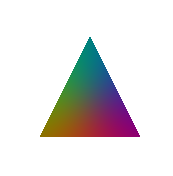Android Question Draw a triangle with color interpolation

Discussion in 'Android Questions' started by Marcob, Aug 30, 2018.

Tags:
1. Given x,y coordinates and color attribute of each vertex of a triangle, does exist a library able to draw the triangle filled with interpolated colors?

Attached Files:

• triangle.png
File size:
8.4 KB
Views:
57
2. Tested in B4J. Layout with an ImageView named ImageView1:

Code:
Sub Process_Globals

Private MainForm As Form

Private xui As XUI

Private ImageView1 As B4XView
End Sub

Sub AppStart (Form1 As Form, Args() As String)
MainForm = Form1
"1"'Load the layout file.
MainForm.Show

Dim pnl As B4XView = xui.CreatePanel("")
pnl.SetLayoutAnimated(
000100100)

Dim cvs As B4XCanvas
cvs.Initialize(pnl)

Dim Points As List = Array(Array As Int(500), Array As Int(100100), Array As Int(0100))

Dim triangle As B4XPath

Dim p() As Int = Points.Get(0)
triangle.Initialize(p(
0), p(1))
p = Points.Get(
1)
triangle.LineTo(p(
0), p(1))
p = Points.Get(
2)
triangle.LineTo(p(
0), p(1))
cvs.DrawPath(triangle, xui.Color_Black,
True0)

Dim bc As BitmapCreator
bc.Initialize(
100100)
bc.CopyPixelsFromBitmap(cvs.CreateBitmap)

Dim clrs(3As Int = Array As Int(xui.Color_Red, xui.Color_Green, xui.Color_Blue)

Dim argbs(3As ARGBColor

Dim NewColor As ARGBColor
NewColor.a =
255

For i = 0 To 2
bc.ColorToARGB(clrs(i), argbs(i))

Next

Dim dists(3As Float

For y = 0 To bc.mHeight - 1

For x = 0 To bc.mWidth - 1

If bc.IsTransparent(x, y) = False Then

For i = 0 To 2
dists(i) = CalcDistance(Points.Get(i), x, y)

Next

Dim total As Float = dists(0) + dists(1) + dists(2)
NewColor.r =
0
NewColor.g =
0
NewColor.b =
0

For i = 0 To 2
NewColor.r = NewColor.r + argbs(i).r * dists(i) / total
NewColor.g = NewColor.g + argbs(i).g * dists(i) / total
NewColor.b = NewColor.b + argbs(i).b * dists(i) / total

Next
bc.SetARGB(x, y, NewColor)

End If

Next

Next
ImageView1.SetBitmap(bc.Bitmap)
End Sub

Private Sub CalcDistance(From() As Int, ToX As Int, ToY As Int) As Float

Return Power(From(0) - ToX, 2) + Power(From(1) - ToY, 2'Distance ^ 2
End Sub
Depends on XUI, BitmapCreator.It is not exactly the same interpolation. Hopefully it will help you get started.

JordiCP and Peter Simpson like this.
3. Thank you very much.
I'll give it a try as soon as I can.